# Peak Detection

Provides a demonstration of using the peak detection module available in JDSP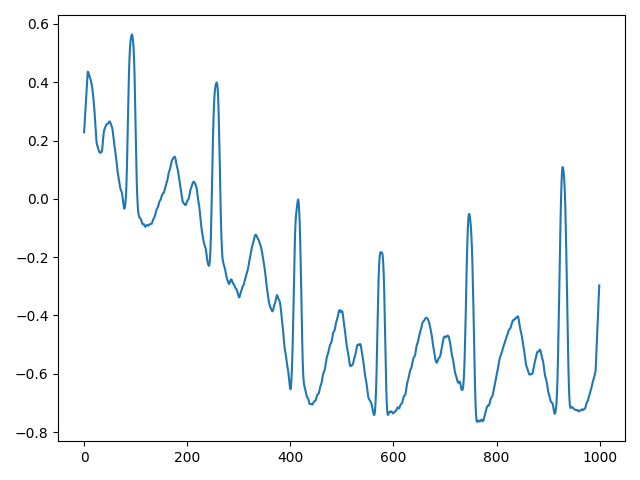## Peak Detection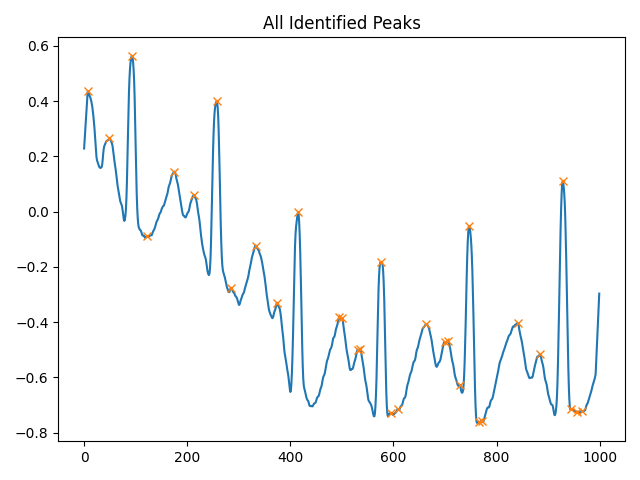##### All Detected Peaks
```                    ```
FindPeak fp = new FindPeak(this.highResSignal);

Peak out = fp.detectPeaks();
int[] peaks = out.getPeaks();

Peak out2 = fp.detectTroughs();
int[] troughs = out2.getPeaks();
```
```

## Peak Filtering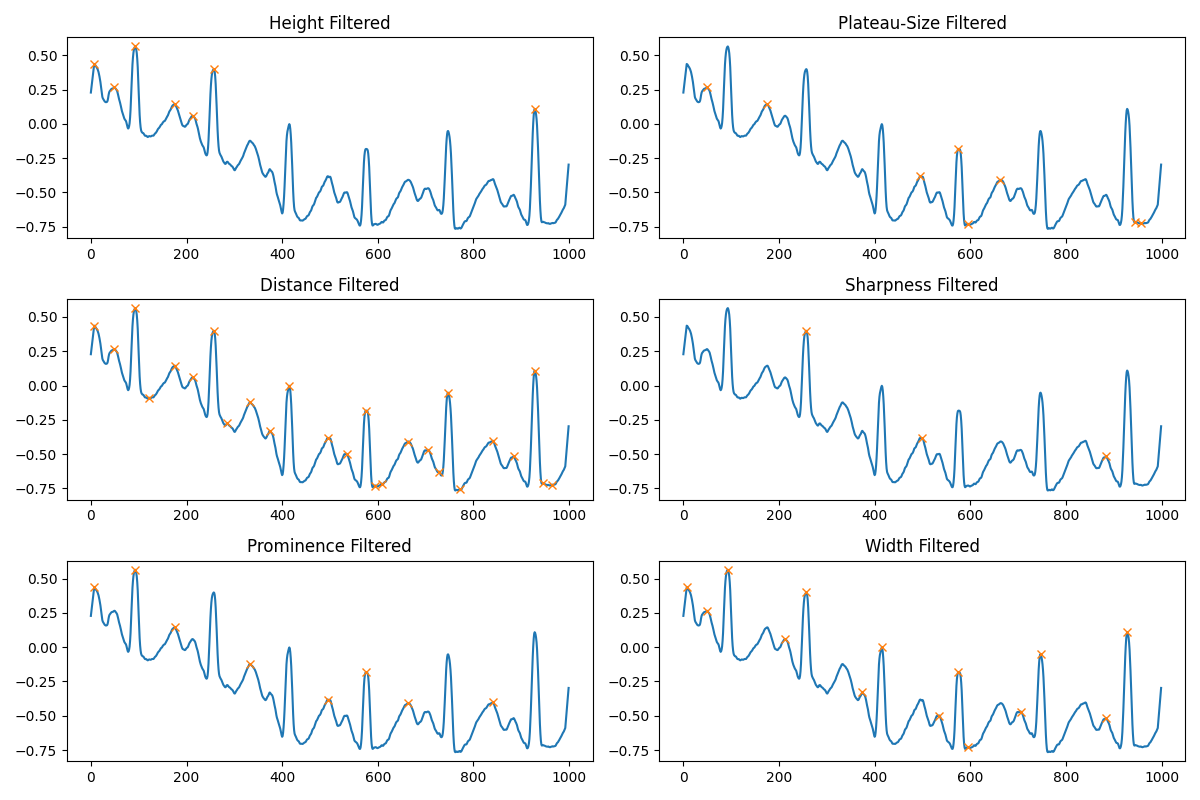#### Plateau Size Filtered

```                    ```
double[] outHeight = out.getHeights(); //To get height of all peaks
double[] height = out.findPeakHeights(peaks); //To calculate height of specific peaks
int[] filteredPeaks1 = out.filterByHeight(0.02, 1.0); //To filter peaks by height
```
```
```                    ```
int[] outPlateau = out.getPlateauSize(); //To get plateau size of all peaks
int[] outPlateau = out.findPlateauSize(peaks); //To calculate plateau size of specific peaks
int[] outFilteredPS1 = out.filterByPlateauSize(2.0, 4.0); //To filter peaks by plateau size
```
```

#### Sharpness Filtered

```                    ```
int[] outDistance = out.getPeakDistance(); //To get distance of all peaks
int[] distance = out.findPeakDistance(peaks); //To calculate distance of specific peaks
int[] outFilteredDistance1 = out.filterByPeakDistance(12); //To filter peaks by distance
```
```
```                    ```
double[][] outSharpness = out.getPeakSharpness(); //To get sharpness of all peaks
double[][] sharpness = out.findPeakSharpness(peaks); //To calculate sharpness of specific peaks
int[] filteredSharp1 = out.filterBySharpness(0.002, 0.005); //To filter peaks by sharpness
```
```

#### Width Filtered

```                    ```
double[][] outPromData = out.getProminenceData(); // To get the prominence, left base and right base
double[] outProminence = out.getProminence(); //To get only prominence of all peaks

double[][] promData = out.findPeakProminence(peaks); // To get the prominence, left base and right base
double[] prominence = promData; //To calculate only prominence of specific peaks

int[] filteredProm1 = out.filterByProminence(0.2, 0.6); //To filter peaks by prominence
```
```
```                    ```
double[][] outWidthData = out.getWidthData(); // To get the width, left base and right base at a relative height of 0.5
double[] outWidth = out.getWidth(); //To get width of all peaks

double[][] widthData = out.findPeakWidth(peaks, 0.5); // To get the width, left base and right base at a relative height of 0.5
double[] width = widthData; //To calculate width of specific peaks

int[] filteredWidth1 = out.filterByWidth(5.0, 20.0); //To filter peaks by width
```
```## Spike Detection (Check Wiki)##### All Detected Spikes
```                    ```
FindPeak fp = new FindPeak(this.highResSignal);
Spike out = fp.getSpikes();

double[] outLeftSpike = out.getLeftSpike();
double[] outRightSpike = out.getRightSpike();
double[] outMeanSpike = out.getMeanSpike();
double[] outMaxSpike = out.getMaxSpike();
double[] outMinSpike = out.getMinSpike();
```
```

## Spike Filtering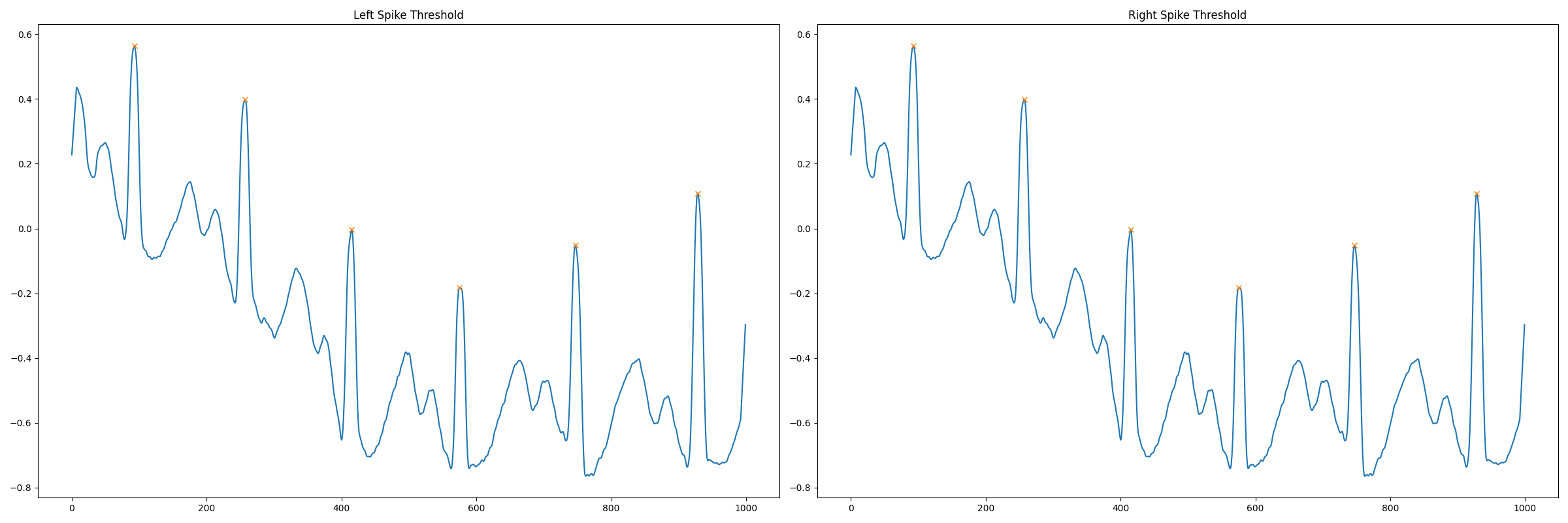#### Right-Spike Filtered

```                    ```
int[] outLeftFilter = out.filterByProperty(0.5, 5.0, "left");
```
```
```                    ```
int[] outRightFilter = out.filterByProperty(0.5, 5.0, "right");
```
```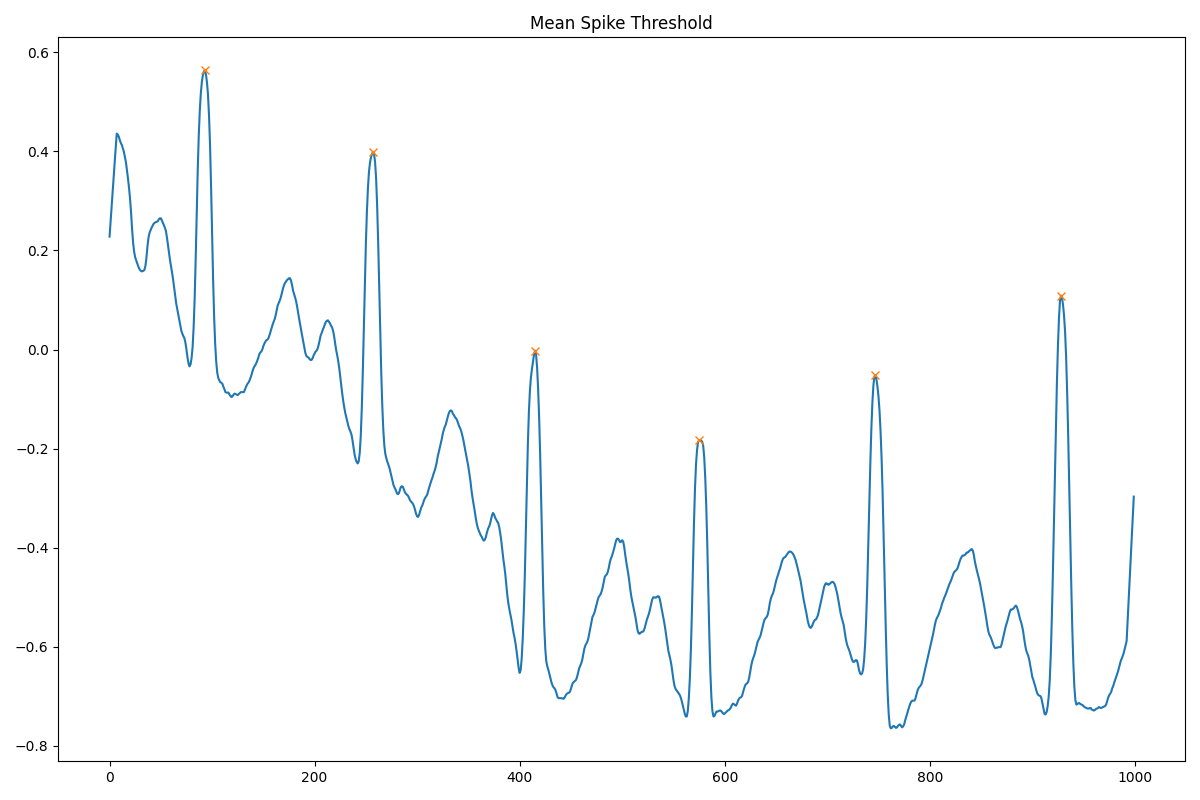#### Mean Filtered

```                ```
int[] outMeanFilter = out.filterByProperty(0.5, 5.0, "mean");
```
```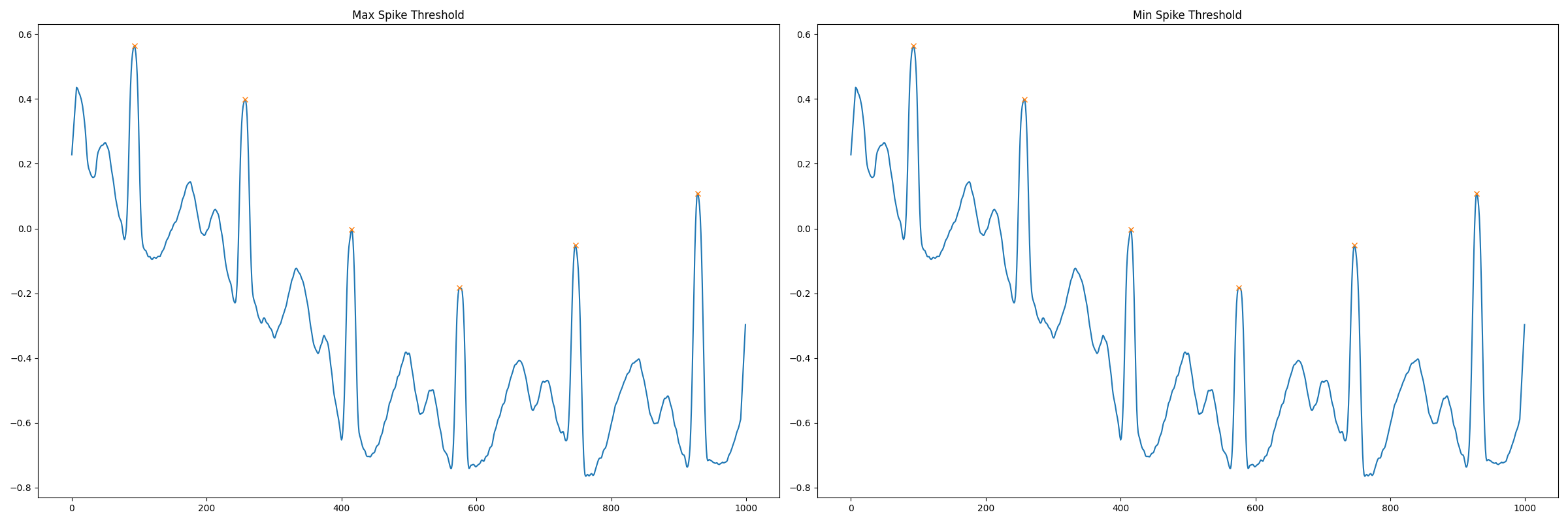#### Min-Spike Filtered

```                ```
int[] outMaxFilter = out.filterByProperty(0.5, 5.0, "max");
```
```
```                ```
int[] outMinFilter = out.filterByProperty(0.5, 5.0, "min");
```
```